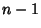## Cyclic Number

A number havingDigits which, when Multiplied by 1, 2, 3, ...,, produces the same digits in a different order. Cyclic numbers are generated by the Unit Fractionswhich have maximal period Decimal Expansions (which meansmust be Prime). The first few numbers which generate cyclic numbers are 7, 17, 19, 23, 29, 47, 59, 61, 97, ... (Sloane's A001913). A much larger generator is 17389.

It has been conjectured, but not yet proven, that an Infinite number of cyclic numbers exist. In fact, the Fraction of Primes which generate cyclic numbers seems to be approximately 3/8. See Yates (1973) for a table of Prime period lengths for Primes. When a cyclic number is multiplied by its generator, the result is a string of 9s. This is a special case of Midy's Theorem.

07=0.142857

17=0.0588235294117647

19=0.052631578947368421

23=0.0434782608695652173913

29=0.0344827586206896551724137931

47=0.0212765957446808510638297872340425531914893617

59=0.0169491525423728813559322033898305084745762711864406779661

61=0.016393442622950819672131147540983606557377049180327868852459

97=0.010309278350515463917525773195876288659793814432989690721649484536082474226804123711340206185567

References

Gardner, M. Ch. 10 in Mathematical Circus: More Puzzles, Games, Paradoxes and Other Mathematical Entertainments from Scientific American. New York: Knopf, 1979.

Guttman, S. ``On Cyclic Numbers.'' Amer. Math. Monthly 44, 159-166, 1934.

Kraitchik, M. ``Cyclic Numbers.'' §3.7 in Mathematical Recreations. New York: W. W. Norton, pp. 75-76, 1942.

Rao, K. S. ``A Note on the Recurring Period of the Reciprocal of an Odd Number.'' Amer. Math. Monthly 62, 484-487, 1955.

Sloane, N. J. A. Sequence A001913/M4353 in ``An On-Line Version of the Encyclopedia of Integer Sequences.'' http://www.research.att.com/~njas/sequences/eisonline.html and Sloane, N. J. A. and Plouffe, S. The Encyclopedia of Integer Sequences. San Diego: Academic Press, 1995.

Yates, S. Primes with Given Period Length. Trondheim, Norway: Universitetsforlaget, 1973.